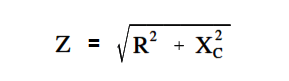# Impedance in RC Circuits

In a capacitive circuit, as in an inductive circuit, impedance is the resultant of phasor addition of R and XC.

The below Equation is the mathematical representation for impedance in an R-C circuit.Example:

A 50 Ω XC and a 60 Ω resistance are in series across a 110V source (Figure 7). Calculate the impedance.Figure 7 : Simple R-C Circuit

Solution:Z = √ (602 + 502)

Z = √6100

Z = 78.1 Ω

Don't Miss Our Updates
Be the first to get exclusive content straight to your email.
We promise not to spam you. You can unsubscribe at any time.